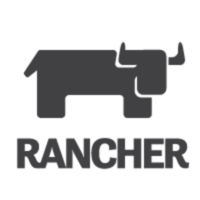# MATLAB vs YAML

MATLAB

446
356
+ 1
22
YAML

167
104
+ 1
0

MATLAB vs YAML: What are the differences?

What is MATLAB? A high-level language and interactive environment for numerical computation, visualization, and programming. Using MATLAB, you can analyze data, develop algorithms, and create models and applications. The language, tools, and built-in math functions enable you to explore multiple approaches and reach a solution faster than with spreadsheets or traditional programming languages, such as C/C++ or Java.

What is YAML? A straightforward machine parsable data serialization format designed for human readability and interaction. A human-readable data-serialization language. It is commonly used for configuration files, but could be used in many applications where data is being stored or transmitted.

MATLAB and YAML can be categorized as "Languages" tools.

According to the StackShare community, MATLAB has a broader approval, being mentioned in 12 company stacks & 23 developers stacks; compared to YAML, which is listed in 5 company stacks and 4 developer stacks.

Pros of MATLAB
Pros of YAML
No pros available

Cons of MATLAB
Cons of YAML
No cons available

## What is MATLAB?

Using MATLAB, you can analyze data, develop algorithms, and create models and applications. The language, tools, and built-in math functions enable you to explore multiple approaches and reach a solution faster than with spreadsheets or traditional programming languages, such as C/C++ or Java.

## What is YAML?

A human-readable data-serialization language. It is commonly used for configuration files, but could be used in many applications where data is being stored or transmitted.
What companies use MATLAB?
What companies use YAML?

What tools integrate with MATLAB?
What tools integrate with YAML?

### Blog Posts

Jun 16, 2020 at 5:38PMRancher Labs

3
504
Jan 29, 2020 at 2:08PMRed Hat, Inc.

11
1783
What are some alternatives to MATLAB and YAML?
R Language
R provides a wide variety of statistical (linear and nonlinear modelling, classical statistical tests, time-series analysis, classification, clustering, ...) and graphical techniques, and is highly extensible.
NumPy
Besides its obvious scientific uses, NumPy can also be used as an efficient multi-dimensional container of generic data. Arbitrary data-types can be defined. This allows NumPy to seamlessly and speedily integrate with a wide variety of databases.
Octave
It is software featuring a high-level programming language, primarily intended for numerical computations. Octave helps in solving linear and nonlinear problems numerically, and for performing other numerical experiments using a language that is mostly compatible with MATLAB.
Tableau
Tableau can help anyone see and understand their data. Connect to almost any database, drag and drop to create visualizations, and share with a click.
Python
Python is a general purpose programming language created by Guido Van Rossum. Python is most praised for its elegant syntax and readable code, if you are just beginning your programming career python suits you best.
See all alternatives
Interest over time
How much does MATLAB cost?
How much does YAML cost?
Pricing unavailable